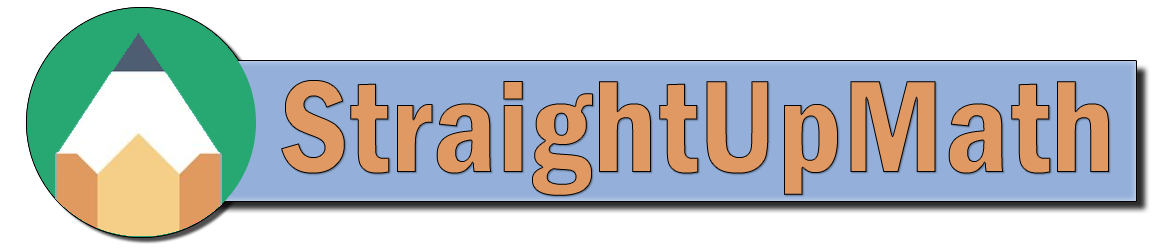Percents - Finding the Whole Number

## Practice

Complete the following.
a)    138 is 30% of what number?
460

b)    329 is 35% of what number?
940

c)    423 is 45% of what number?
940

d)    144 is 30% of what number?
480

e)    10 is 10% of what number?
100

f)    360 is 60% of what number?
600

g)    162 is 90% of what number?
180

h)    250 is 50% of what number?
500

i)    90 is 15% of what number?
600

j)    105 is 15% of what number?
700